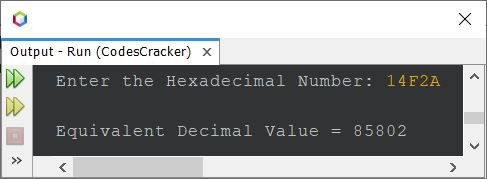# Java Program to Convert Hexadecimal to Decimal

If you're not aware about, how the hexadecimal to decimal conversion takes place, then refer to Hexadecimal to Decimal Conversion. Now let's move on, and create the program for the conversion.

## Hexadecimal to Decimal Conversion in Java

The question is, write a Java program to convert hexadecimal number to decimal. The program given below is its answer:

```import java.util.Scanner;
import java.lang.Math;

public class CodesCracker
{
public static void main(String[] args)
{
int decimal=0, i=0, len, rem;

Scanner s = new Scanner(System.in);

len--;
while(len>=0)
{
if(rem>=48 && rem<=57)
rem = rem-48;
else if(rem>=65 && rem<=70)
rem = rem-55;
else if(rem>=97 && rem<=102)
rem = rem-87;
else
{
return;
}
decimal = (int) (decimal + (rem*Math.pow(16, i)));
i++;
len--;
}

System.out.println("\nEquivalent Decimal Value = " +decimal);
}
}```

The sample run with user input 14F2A as hexadecimal number to convert and print its equivalent decimal value, is shown in the snapshot given below:The ASCII values of A-Z are 65-90 whereas the ASCII values of a-z are 97-122.

That is, the ASCII value of 'A' is 65, 'B' is 66, and so on. Therefore, from above program, the following block of code:

```if(rem>=48 && rem<=57)
rem = rem-48;
else if(rem>=65 && rem<=70)
rem = rem-55;
else if(rem>=97 && rem<=102)
rem = rem-87;```

can also be replaced with, or written as, the block of code given below:

```if(rem>='0' && rem<='9')
rem = rem-48;
else if(rem>='A' && rem<='F')
rem = rem-55;
else if(rem>='a' && rem<='f')
rem = rem-87;```

And if you do not want to use the Math.pow() method, then replace the following statement:

`decimal = (int) (decimal + (rem*Math.pow(16, i)));`

with the block of code given below:

```int m=1;
for(int k=1; k<=i; k++)
m *= 16;
decimal = (int) (decimal + (rem*m));```

#### Same Program in Other Languages

Java Online Test

« Previous Program Next Program »